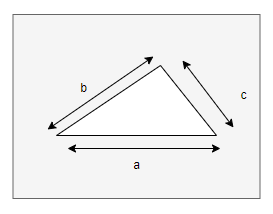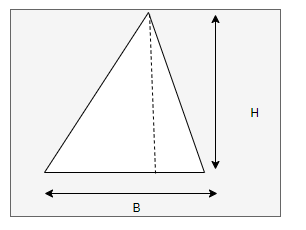# Area of a Triangle Calculator

## Area of a Triangle Calculator(Heron Formula)Note
• Enter the values of Sides of the triangle
• Click on the calculate button to get the value of Area

Formula And concept used
if a, b,c are sides of the triangle,then Area is given $A = \sqrt {s(s-a)(s-b)(s-c)}$
Where
$s = \frac {a + b + c}{2}$

### Area of a Triangle Calculator(Heron Formula)

Example of Few questions where you can use this Area of a Triangle Calculator(Heron Formula Calculator)
1. Find the Area of a Triangle.
i. 3, 6 , 4
ii. 30, 25 , 40

## How Calculator works

• First value of s is calculated as
$s= \frac {a+b+c}{2}$
• Now area is calculated using the formula
$A = \sqrt {s(s-a)(s-b)(s-c)}$

## Area of a Triangle Calculator Using Height and BaseNote
• Enter the values of Height and Base of the triangle
• Click on the calculate button to get the value of Area

Formula And concept used
if B and H are Base and Height of the triangle,then Area is given $A = \frac {1}{2} B \times H$

### Area of a Triangle Calculator Using Height and Base

Example of Few questions where you can use this Area of a Triangle Calculator Using Base And Height
1. Find the Area of a Triangle.
i. Base=10 cm, Height =8 cm
ii. Base=15 cm, Height =20 cm